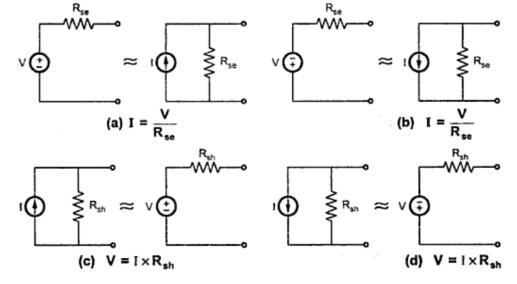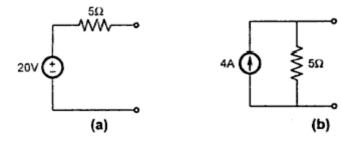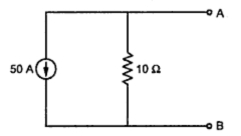### Source Transformation

Consider a practical voltage source shown in the Fig. 1.(a) having internal resistance Rse, connected to the load having resistance RL .Fig. 1(a
Now we can replace voltage source by equivalent current source.
Key point : The tow sources are said to be equivalent, if they supply equal load current to the load, with same load connected across its terminals.
The current delivered in above case by voltage source is,
I = V/ (Rse  + RL ) , Rse  and R are in series             ..............(1)
If it is to be replaced by a current source then load current must be V/(Rse  +RL ).
Consider an equivalent current source shown in the Fig. 1(b).Fig 1 (b) .
The total current is T.
Both the resistances will take current proportional to their values.
From the current division in parallel circuit we can write,
............(2)
Now this I and V/(Rsh  +R )must be same, so equating (1) and (2),
Let internal resistance be,  Rse  =  Rsh  = R  say.
Then, V= I x Rsh  = I x R
or       I= V/Rsh
...              I = V/R = V/Rse
Key point : If voltage source is converted to current source, then current source I = V/ Rse with parallel internal resistance equal to Rse .
Key point : If current source is converted to voltage source, the voltage source V = I Rsh  with sereis internal resistance equal to Rsh .
The direction of current of equivalent circuit source is always from -ve to +ve, internal to the source. While converting current source to voltage source, polarities of voltage is always as +ve terminal at top of arrow and -ve terminal at bottom of arrow, as direction of current is from -ve to +ve, internal to the source. This ensure that current flows from positive to negative terminal in the external circuit.
Note the direction of transformed sources, shown in the Fig. 2(a), (b), (c) and (d).Fig. 2
Example 1 : Transform a voltage source of 20 volts with an internal resistance of 5 Ω to a current source.
Solution : Refer to the Fig. 3(a)Fig. 3
The current of current source is, I = V/Rse  = 20/5 = 4 A with internal parallel resistance same as Rse .
Equivalent current source is as shown in the Fig. 3(b).
Example 2 : Convert the given current source of 50 A with internal resistance of 10 Ω to the equivalent voltage source.Fig. 4
Solution : The given values are,          I= 50 A and  Rsh = 10 Ω.
For the equivalent voltage source,
V= I x = 50 x 10 = 500 V
Rse = Rsh = 10 Ω in series.
The equivalent voltage source is shown in the Fig. 4(a).Fig 4 (a)
Note the polarities of voltage source, which are such that +ve at top of arrow and -ve at bottom.